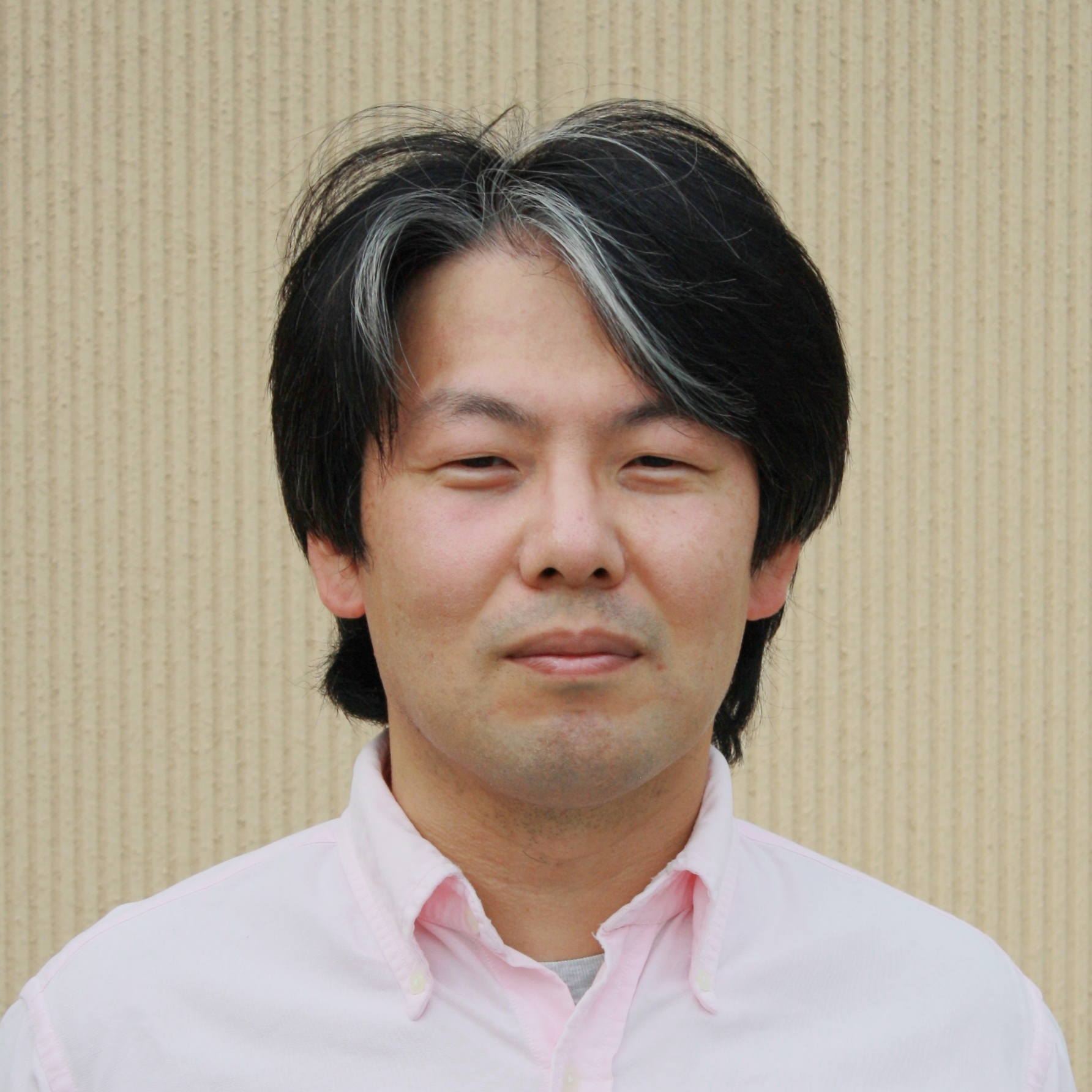## INOGUCHI Jun-ichiProfessor

### Secrets of Shapes

Department of Mathematics, MathematicsTheme Geometric study of Differential equations, Thurston Geometry, Geometric Shape Generation Field Geometry Keyword Lie group, Lie algebra, Homogeneous space, Hamiltonian system, Contact structure, Thurston Geometry

#### Introduction of Research

1) Differential geometric study of differential equations which posses large symmetry groups:
Those differential equations are so-called integrable systems (derived from differential geometry).

2) Differential geometric study of model spaces of 3-dimensional geometry:
According to Thurston, there are eight model spaces of 3-dimensional geometry. The model spaces of Nilgeometry, SL-geometry and Solvgeometry have natural contact structures compatible to the geometric structures. I have been studying these model spaces by virtue of contact structure and homogenous geometry.

#### Representative Achievements

Magnetic Jacobi fields in 3-dimensional Sasakian space forms, J. Inoguchi and M. I. Munteanu, The Journal of Geometric Analysis, 32, Article number 96 (2022)
A loop group method for affine harmonic maps into Lie groups, J.F. Dorfmeister, J. Inoguchi and S.-P. Kobayashi, Advances in Mathematics 298, 207-253 (2016)
Constant mean curvature surfaces in hyperbolic 3-space via loop groups, J.F. Dorfmeister, J. Inoguchi and S.-P. Kobayashi, J. Reine Angew. Math. 686, 1-36 (2014).
Log-aesthetic curves: Similarity geometry, integrable discretization and variational principles, J. Inoguchi, Y. Jikumaru, K. Kajiwara, K. T. Miura, W. K. Schief, Computer Aided Geometric Design 105 (2023) Article Number 102233#### Belongs